Скачать презентацию Fundamentals of General Organic and Biological Chemistry 6

9081d8033e87bc4b0597ed0b6d09d275.ppt

• Количество слайдов: 45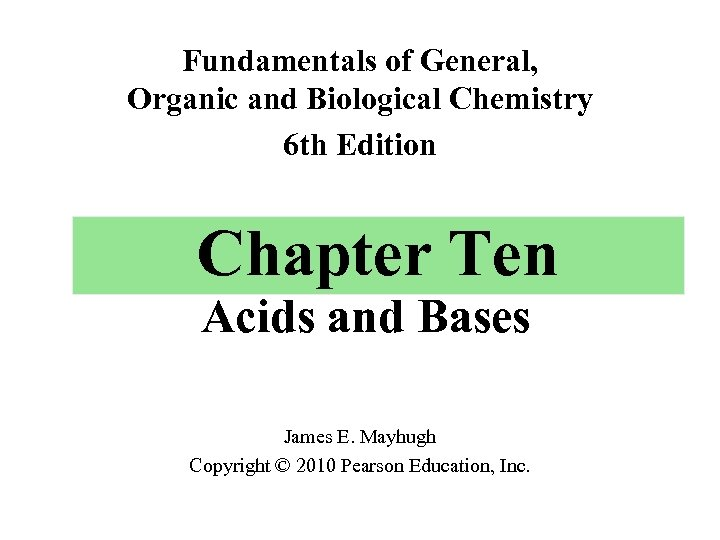Fundamentals of General, Organic and Biological Chemistry 6 th Edition Chapter Ten Acids and Bases James E. Mayhugh Copyright © 2010 Pearson Education, Inc.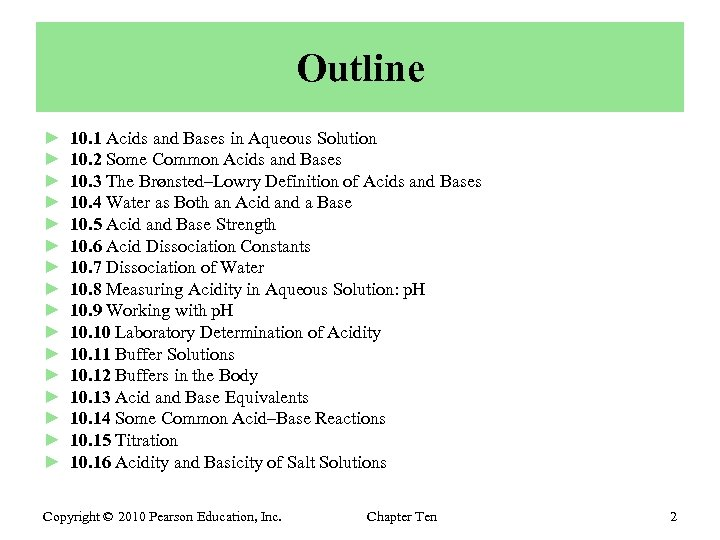Outline ► ► ► ► 10. 1 Acids and Bases in Aqueous Solution 10. 2 Some Common Acids and Bases 10. 3 The Brønsted–Lowry Definition of Acids and Bases 10. 4 Water as Both an Acid and a Base 10. 5 Acid and Base Strength 10. 6 Acid Dissociation Constants 10. 7 Dissociation of Water 10. 8 Measuring Acidity in Aqueous Solution: p. H 10. 9 Working with p. H 10. 10 Laboratory Determination of Acidity 10. 11 Buffer Solutions 10. 12 Buffers in the Body 10. 13 Acid and Base Equivalents 10. 14 Some Common Acid–Base Reactions 10. 15 Titration 10. 16 Acidity and Basicity of Salt Solutions Copyright © 2010 Pearson Education, Inc. Chapter Ten 2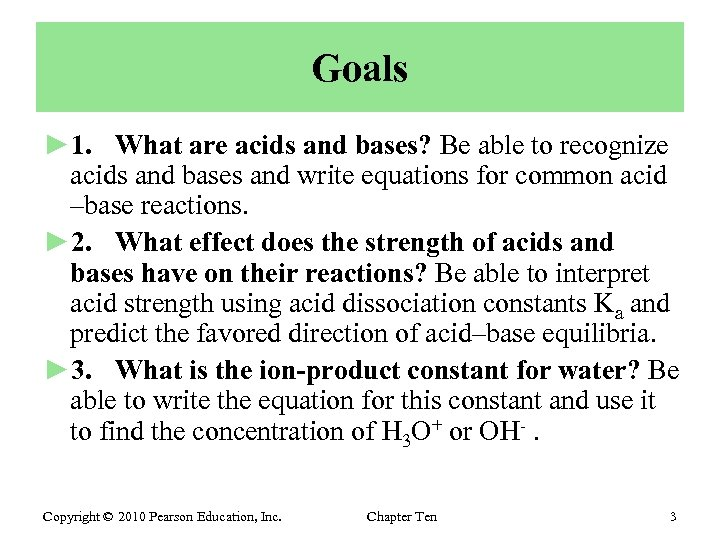Goals ► 1. What are acids and bases? Be able to recognize acids and bases and write equations for common acid –base reactions. ► 2. What effect does the strength of acids and bases have on their reactions? Be able to interpret acid strength using acid dissociation constants Ka and predict the favored direction of acid–base equilibria. ► 3. What is the ion-product constant for water? Be able to write the equation for this constant and use it to find the concentration of H 3 O+ or OH-. Copyright © 2010 Pearson Education, Inc. Chapter Ten 3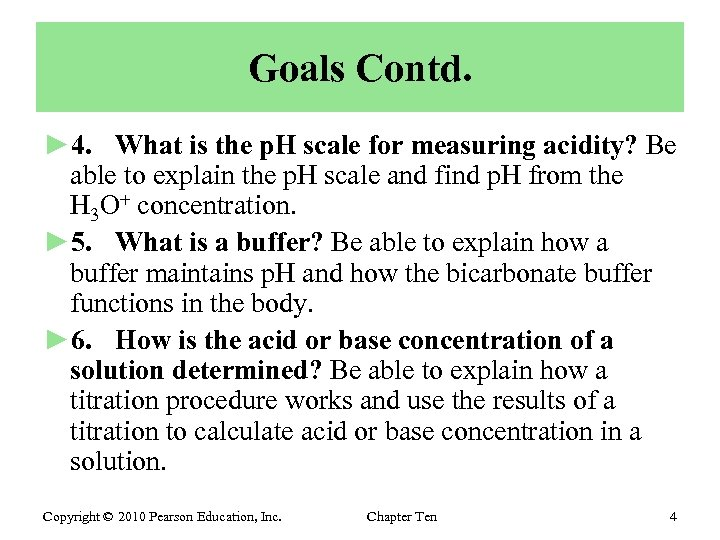Goals Contd. ► 4. What is the p. H scale for measuring acidity? Be able to explain the p. H scale and find p. H from the H 3 O+ concentration. ► 5. What is a buffer? Be able to explain how a buffer maintains p. H and how the bicarbonate buffer functions in the body. ► 6. How is the acid or base concentration of a solution determined? Be able to explain how a titration procedure works and use the results of a titration to calculate acid or base concentration in a solution. Copyright © 2010 Pearson Education, Inc. Chapter Ten 4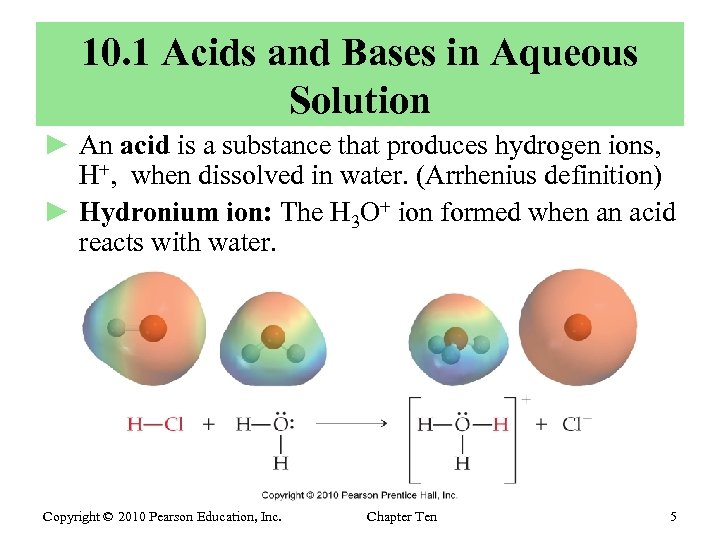10. 1 Acids and Bases in Aqueous Solution ► An acid is a substance that produces hydrogen ions, H+, when dissolved in water. (Arrhenius definition) ► Hydronium ion: The H 3 O+ ion formed when an acid reacts with water. Copyright © 2010 Pearson Education, Inc. Chapter Ten 5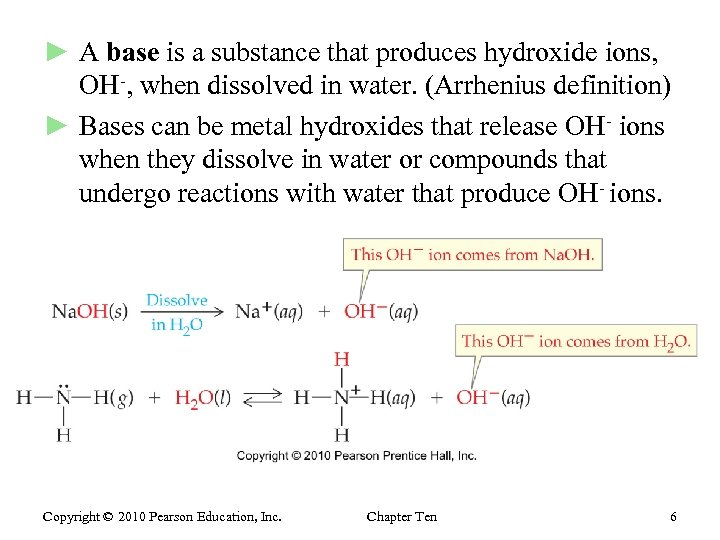► A base is a substance that produces hydroxide ions, OH-, when dissolved in water. (Arrhenius definition) ► Bases can be metal hydroxides that release OH- ions when they dissolve in water or compounds that undergo reactions with water that produce OH- ions. Copyright © 2010 Pearson Education, Inc. Chapter Ten 6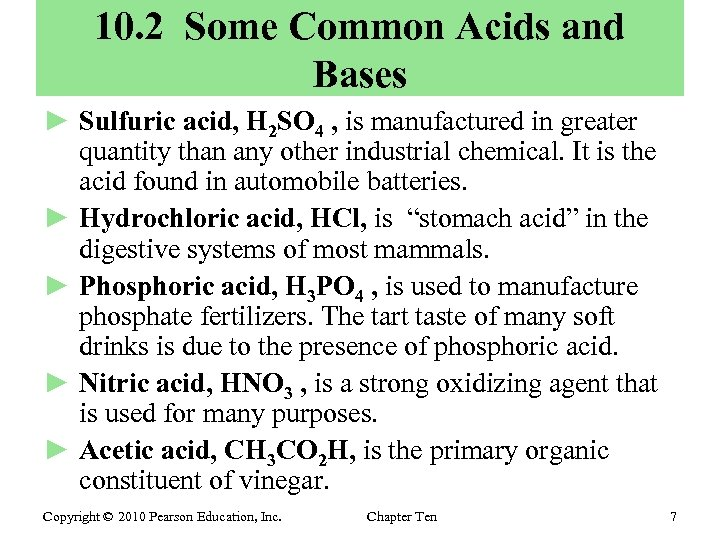10. 2 Some Common Acids and Bases ► Sulfuric acid, H 2 SO 4 , is manufactured in greater quantity than any other industrial chemical. It is the acid found in automobile batteries. ► Hydrochloric acid, HCl, is “stomach acid” in the digestive systems of most mammals. ► Phosphoric acid, H 3 PO 4 , is used to manufacture phosphate fertilizers. The tart taste of many soft drinks is due to the presence of phosphoric acid. ► Nitric acid, HNO 3 , is a strong oxidizing agent that is used for many purposes. ► Acetic acid, CH 3 CO 2 H, is the primary organic constituent of vinegar. Copyright © 2010 Pearson Education, Inc. Chapter Ten 7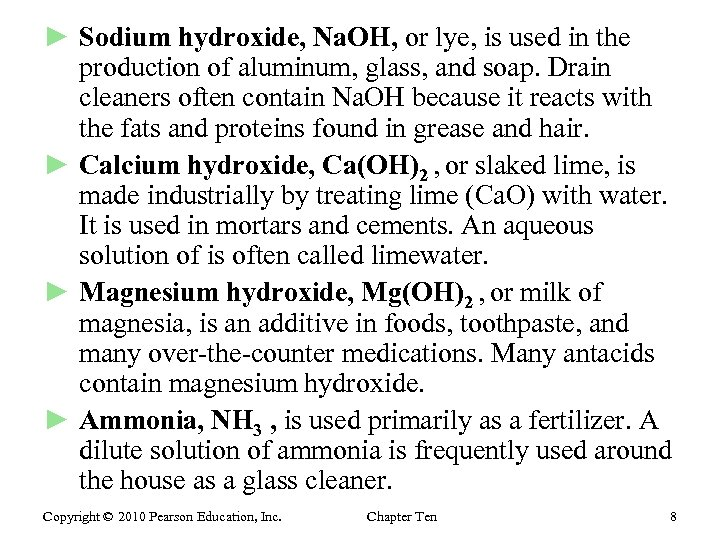► Sodium hydroxide, Na. OH, or lye, is used in the production of aluminum, glass, and soap. Drain cleaners often contain Na. OH because it reacts with the fats and proteins found in grease and hair. ► Calcium hydroxide, Ca(OH)2 , or slaked lime, is made industrially by treating lime (Ca. O) with water. It is used in mortars and cements. An aqueous solution of is often called limewater. ► Magnesium hydroxide, Mg(OH)2 , or milk of magnesia, is an additive in foods, toothpaste, and many over-the-counter medications. Many antacids contain magnesium hydroxide. ► Ammonia, NH 3 , is used primarily as a fertilizer. A dilute solution of ammonia is frequently used around the house as a glass cleaner. Copyright © 2010 Pearson Education, Inc. Chapter Ten 8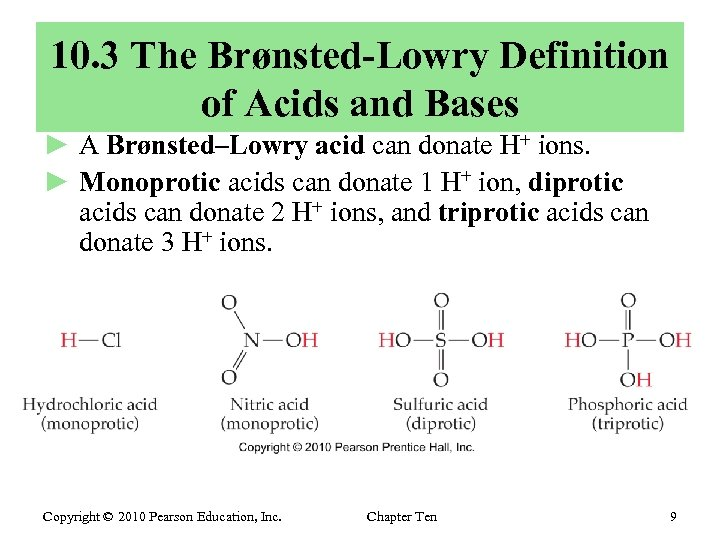10. 3 The Brønsted-Lowry Definition of Acids and Bases ► A Brønsted–Lowry acid can donate H+ ions. ► Monoprotic acids can donate 1 H+ ion, diprotic acids can donate 2 H+ ions, and triprotic acids can donate 3 H+ ions. Copyright © 2010 Pearson Education, Inc. Chapter Ten 9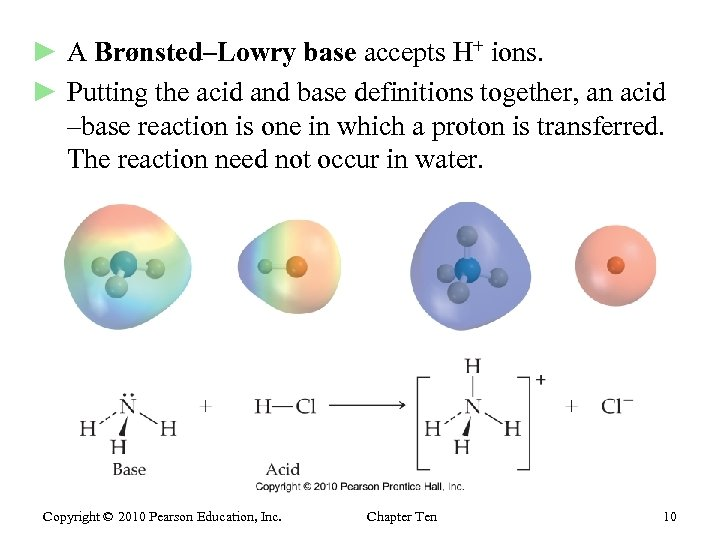► A Brønsted–Lowry base accepts H+ ions. ► Putting the acid and base definitions together, an acid –base reaction is one in which a proton is transferred. The reaction need not occur in water. Copyright © 2010 Pearson Education, Inc. Chapter Ten 10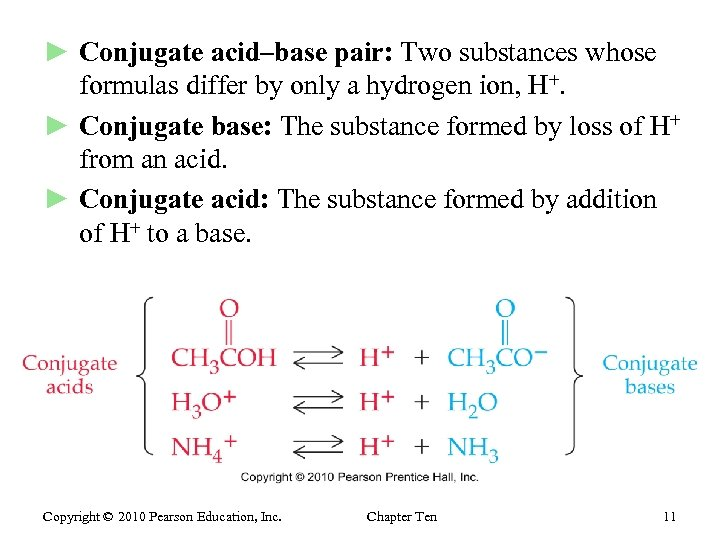► Conjugate acid–base pair: Two substances whose formulas differ by only a hydrogen ion, H+. ► Conjugate base: The substance formed by loss of H+ from an acid. ► Conjugate acid: The substance formed by addition of H+ to a base. Copyright © 2010 Pearson Education, Inc. Chapter Ten 11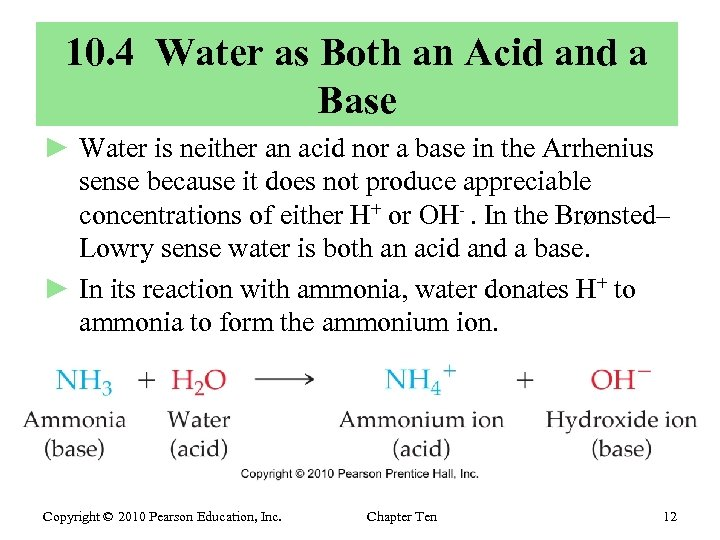10. 4 Water as Both an Acid and a Base ► Water is neither an acid nor a base in the Arrhenius sense because it does not produce appreciable concentrations of either H+ or OH-. In the Brønsted– Lowry sense water is both an acid and a base. ► In its reaction with ammonia, water donates H+ to ammonia to form the ammonium ion. Copyright © 2010 Pearson Education, Inc. Chapter Ten 12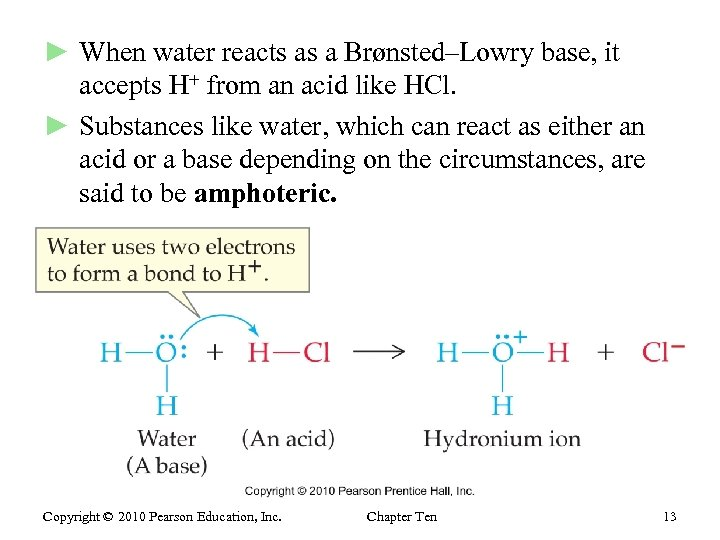► When water reacts as a Brønsted–Lowry base, it accepts H+ from an acid like HCl. ► Substances like water, which can react as either an acid or a base depending on the circumstances, are said to be amphoteric. Copyright © 2010 Pearson Education, Inc. Chapter Ten 13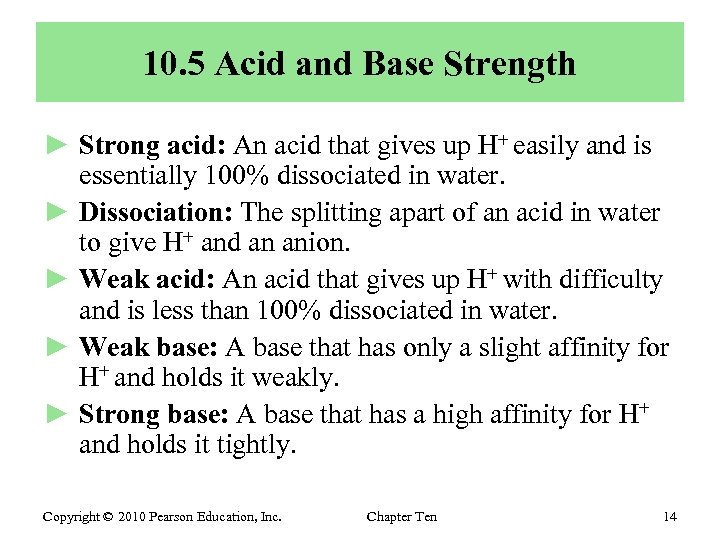10. 5 Acid and Base Strength ► Strong acid: An acid that gives up H+ easily and is essentially 100% dissociated in water. ► Dissociation: The splitting apart of an acid in water to give H+ and an anion. ► Weak acid: An acid that gives up H+ with difficulty and is less than 100% dissociated in water. ► Weak base: A base that has only a slight affinity for H+ and holds it weakly. ► Strong base: A base that has a high affinity for H+ and holds it tightly. Copyright © 2010 Pearson Education, Inc. Chapter Ten 14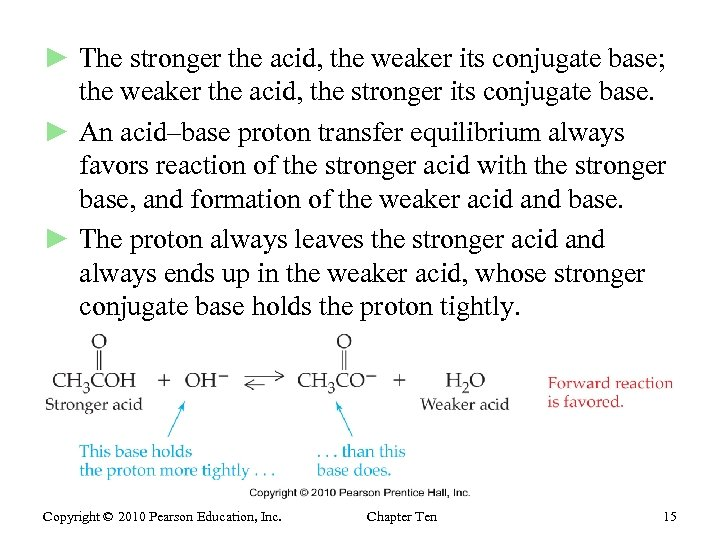► The stronger the acid, the weaker its conjugate base; the weaker the acid, the stronger its conjugate base. ► An acid–base proton transfer equilibrium always favors reaction of the stronger acid with the stronger base, and formation of the weaker acid and base. ► The proton always leaves the stronger acid and always ends up in the weaker acid, whose stronger conjugate base holds the proton tightly. Copyright © 2010 Pearson Education, Inc. Chapter Ten 15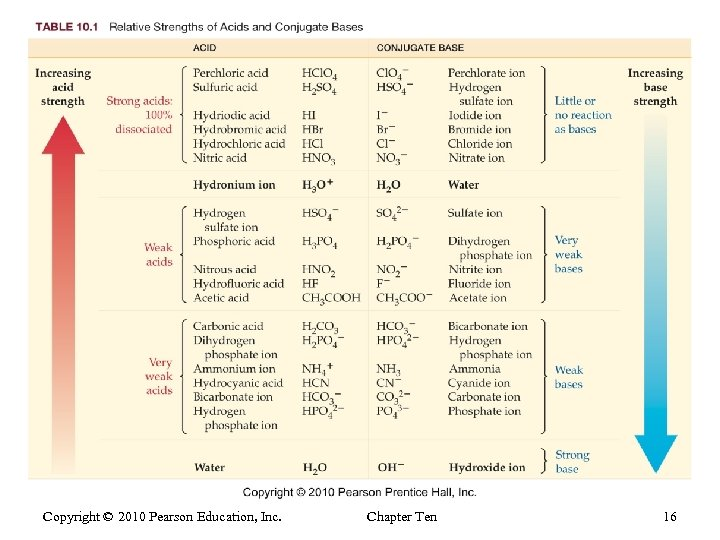Copyright © 2010 Pearson Education, Inc. Chapter Ten 16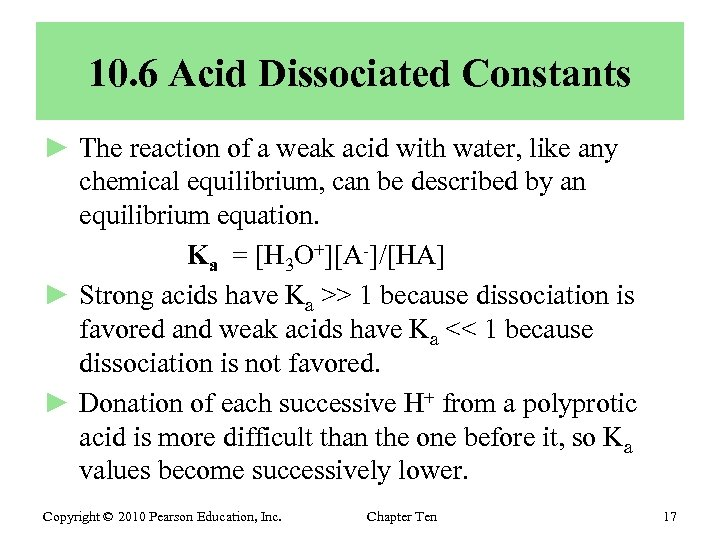10. 6 Acid Dissociated Constants ► The reaction of a weak acid with water, like any chemical equilibrium, can be described by an equilibrium equation. Ka = [H 3 O+][A-]/[HA] ► Strong acids have Ka >> 1 because dissociation is favored and weak acids have Ka << 1 because dissociation is not favored. ► Donation of each successive H+ from a polyprotic acid is more difficult than the one before it, so Ka values become successively lower. Copyright © 2010 Pearson Education, Inc. Chapter Ten 17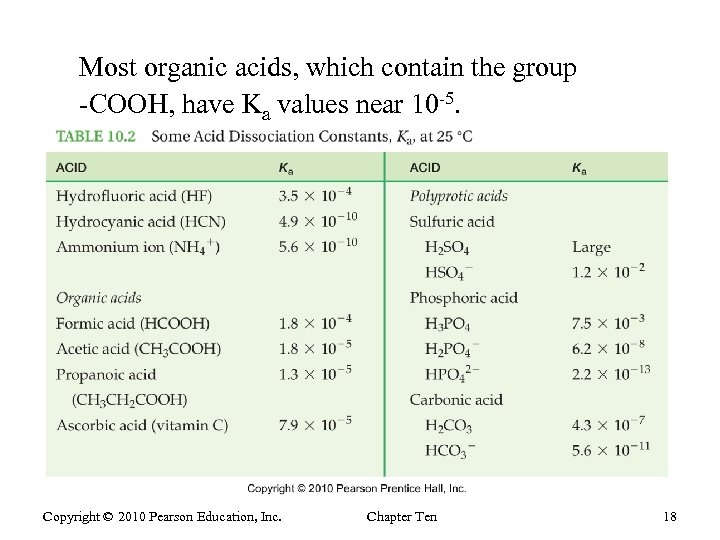Most organic acids, which contain the group -COOH, have Ka values near 10 -5. Copyright © 2010 Pearson Education, Inc. Chapter Ten 18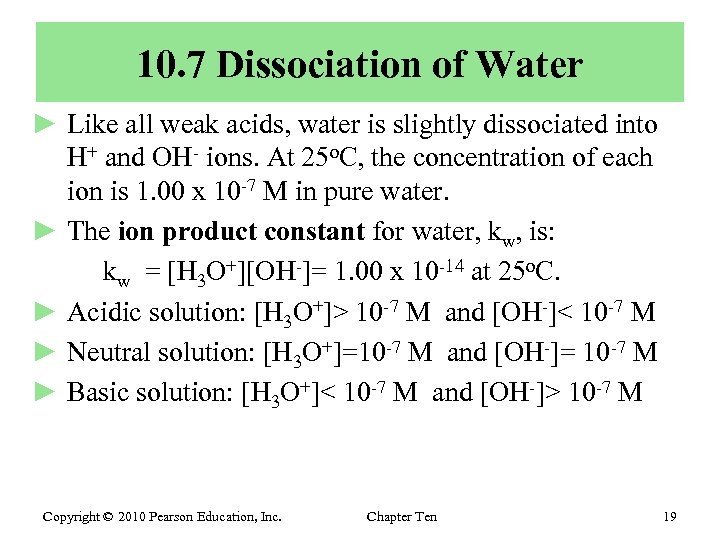10. 7 Dissociation of Water ► Like all weak acids, water is slightly dissociated into H+ and OH- ions. At 25 o. C, the concentration of each ion is 1. 00 x 10 -7 M in pure water. ► The ion product constant for water, kw, is: kw = [H 3 O+][OH-]= 1. 00 x 10 -14 at 25 o. C. ► Acidic solution: [H 3 O+]> 10 -7 M and [OH-]< 10 -7 M ► Neutral solution: [H 3 O+]=10 -7 M and [OH-]= 10 -7 M ► Basic solution: [H 3 O+]< 10 -7 M and [OH-]> 10 -7 M Copyright © 2010 Pearson Education, Inc. Chapter Ten 19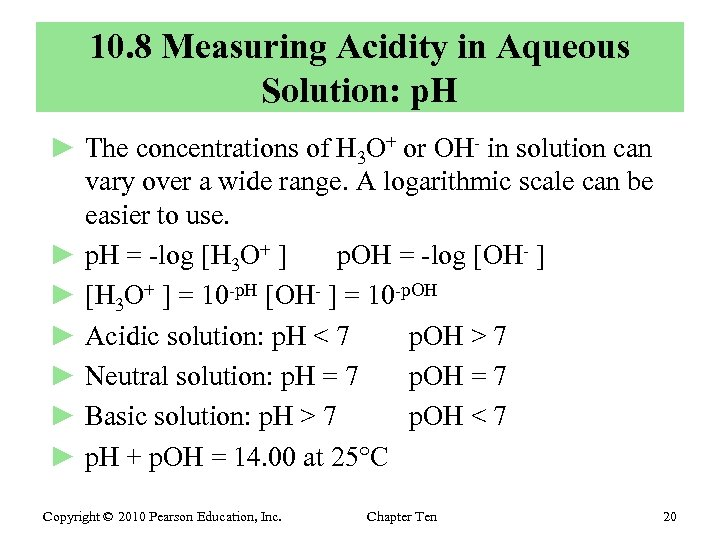10. 8 Measuring Acidity in Aqueous Solution: p. H ► The concentrations of H 3 O+ or OH- in solution can vary over a wide range. A logarithmic scale can be easier to use. ► p. H = -log [H 3 O+ ] p. OH = -log [OH- ] ► [H 3 O+ ] = 10 -p. H [OH- ] = 10 -p. OH ► Acidic solution: p. H < 7 p. OH > 7 ► Neutral solution: p. H = 7 p. OH = 7 ► Basic solution: p. H > 7 p. OH < 7 ► p. H + p. OH = 14. 00 at 25 C Copyright © 2010 Pearson Education, Inc. Chapter Ten 20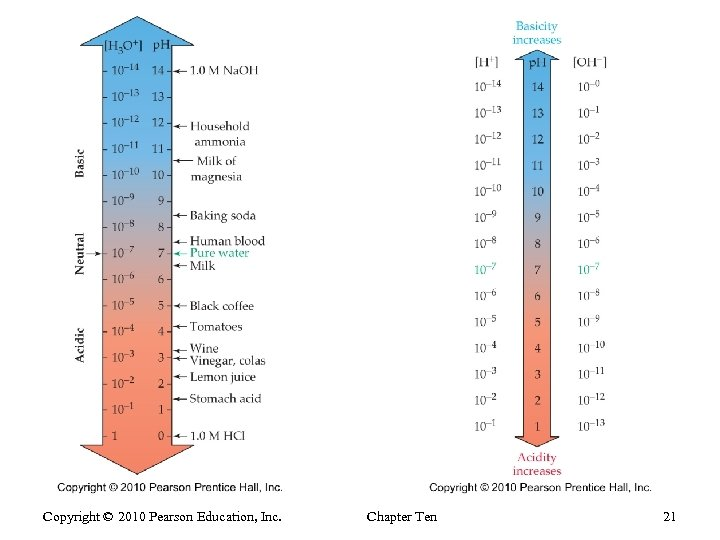Copyright © 2010 Pearson Education, Inc. Chapter Ten 21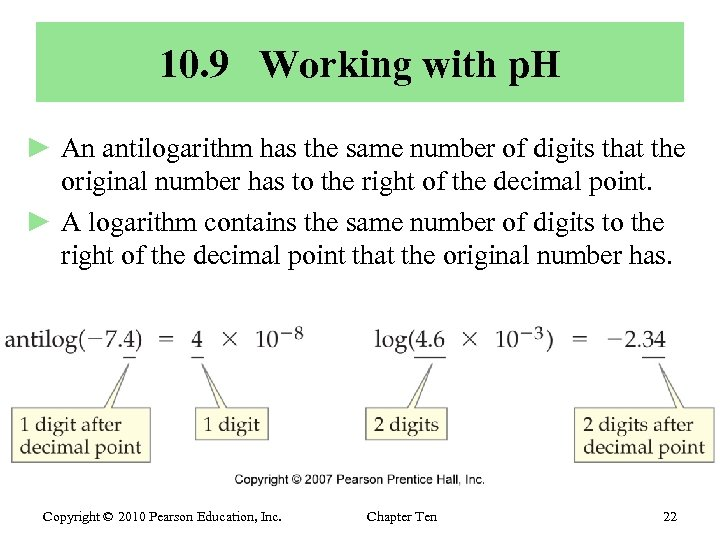10. 9 Working with p. H ► An antilogarithm has the same number of digits that the original number has to the right of the decimal point. ► A logarithm contains the same number of digits to the right of the decimal point that the original number has. Copyright © 2010 Pearson Education, Inc. Chapter Ten 22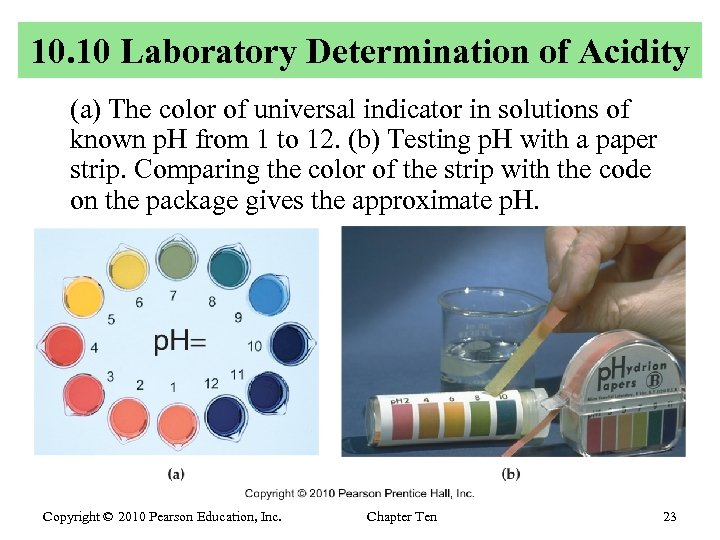10. 10 Laboratory Determination of Acidity (a) The color of universal indicator in solutions of known p. H from 1 to 12. (b) Testing p. H with a paper strip. Comparing the color of the strip with the code on the package gives the approximate p. H. Copyright © 2010 Pearson Education, Inc. Chapter Ten 23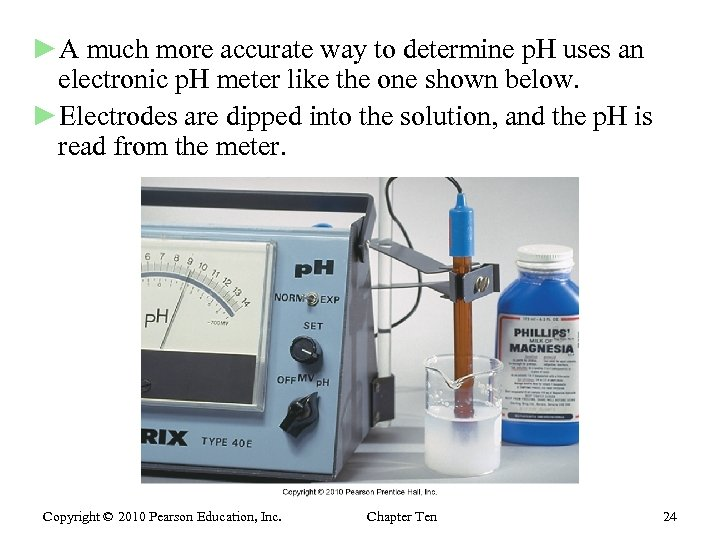►A much more accurate way to determine p. H uses an electronic p. H meter like the one shown below. ►Electrodes are dipped into the solution, and the p. H is read from the meter. Copyright © 2010 Pearson Education, Inc. Chapter Ten 24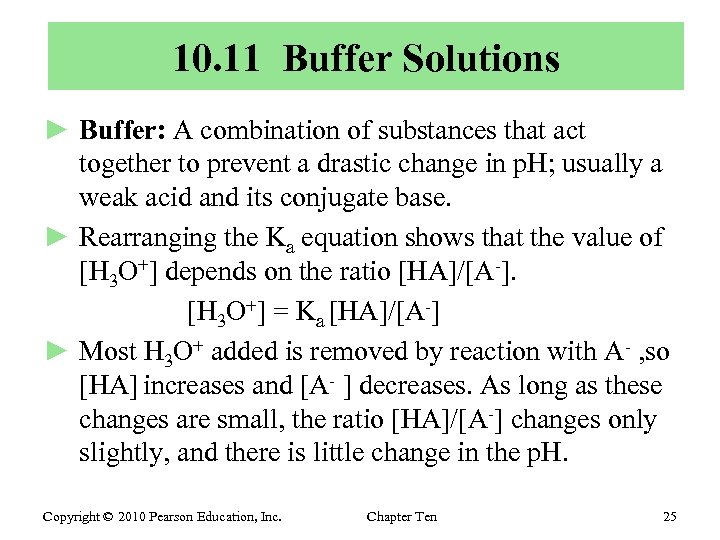10. 11 Buffer Solutions ► Buffer: A combination of substances that act together to prevent a drastic change in p. H; usually a weak acid and its conjugate base. ► Rearranging the Ka equation shows that the value of [H 3 O+] depends on the ratio [HA]/[A-]. [H 3 O+] = Ka [HA]/[A-] ► Most H 3 O+ added is removed by reaction with A- , so [HA] increases and [A- ] decreases. As long as these changes are small, the ratio [HA]/[A-] changes only slightly, and there is little change in the p. H. Copyright © 2010 Pearson Education, Inc. Chapter Ten 25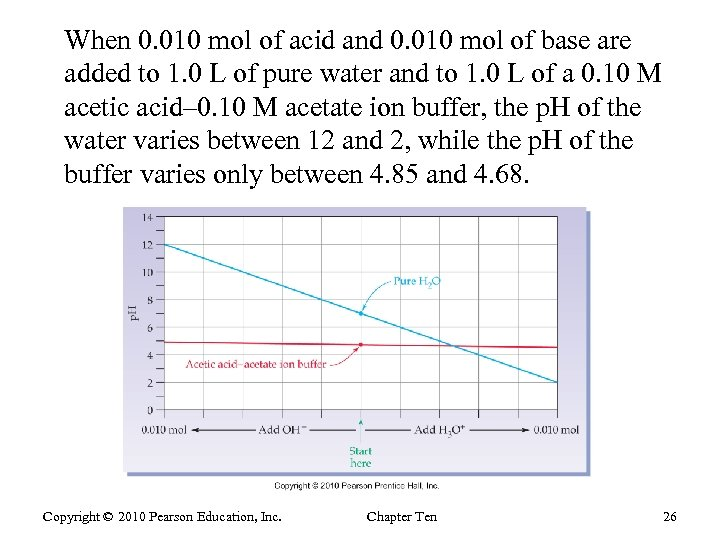When 0. 010 mol of acid and 0. 010 mol of base are added to 1. 0 L of pure water and to 1. 0 L of a 0. 10 M acetic acid– 0. 10 M acetate ion buffer, the p. H of the water varies between 12 and 2, while the p. H of the buffer varies only between 4. 85 and 4. 68. Copyright © 2010 Pearson Education, Inc. Chapter Ten 26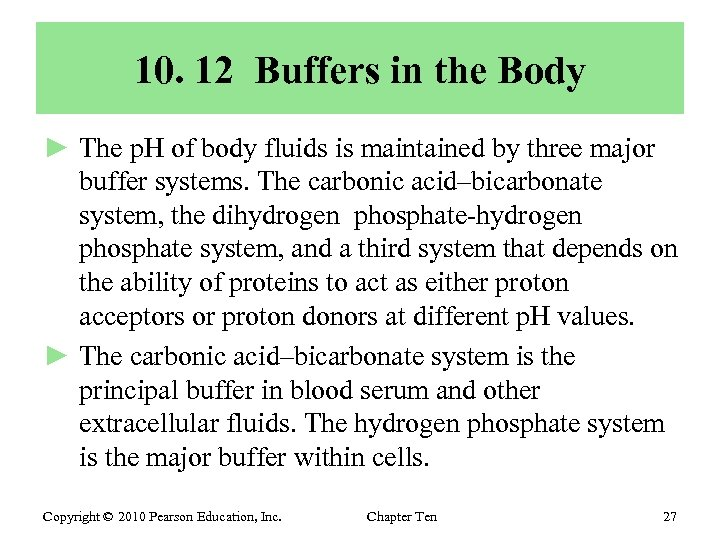10. 12 Buffers in the Body ► The p. H of body fluids is maintained by three major buffer systems. The carbonic acid–bicarbonate system, the dihydrogen phosphate-hydrogen phosphate system, and a third system that depends on the ability of proteins to act as either proton acceptors or proton donors at different p. H values. ► The carbonic acid–bicarbonate system is the principal buffer in blood serum and other extracellular fluids. The hydrogen phosphate system is the major buffer within cells. Copyright © 2010 Pearson Education, Inc. Chapter Ten 27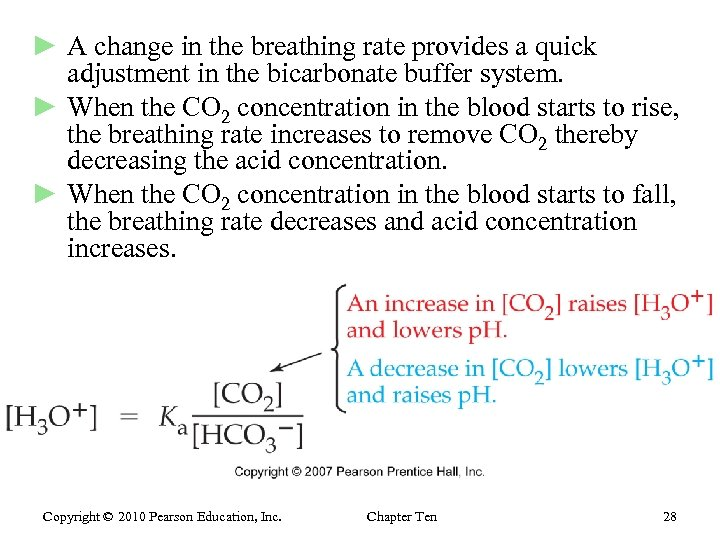► A change in the breathing rate provides a quick adjustment in the bicarbonate buffer system. ► When the CO 2 concentration in the blood starts to rise, the breathing rate increases to remove CO 2 thereby decreasing the acid concentration. ► When the CO 2 concentration in the blood starts to fall, the breathing rate decreases and acid concentration increases. Copyright © 2010 Pearson Education, Inc. Chapter Ten 28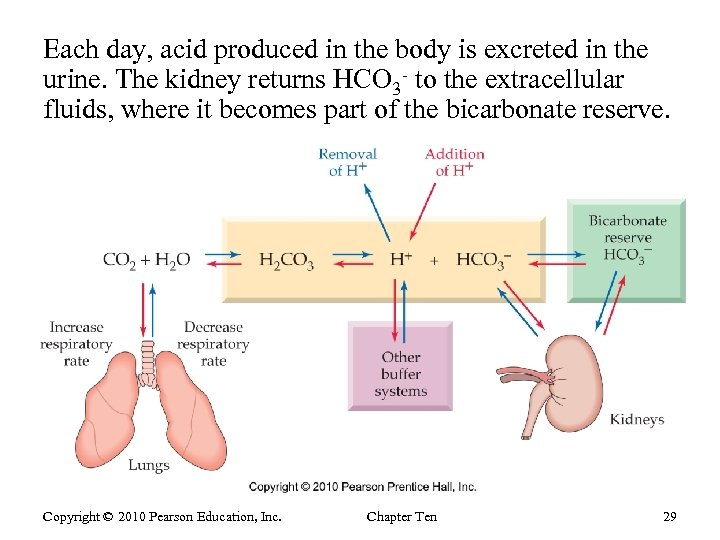Each day, acid produced in the body is excreted in the urine. The kidney returns HCO 3 - to the extracellular fluids, where it becomes part of the bicarbonate reserve. Copyright © 2010 Pearson Education, Inc. Chapter Ten 29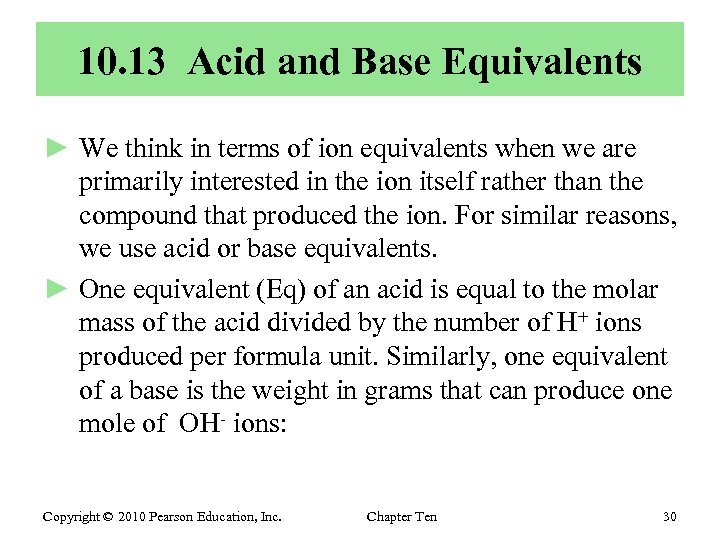10. 13 Acid and Base Equivalents ► We think in terms of ion equivalents when we are primarily interested in the ion itself rather than the compound that produced the ion. For similar reasons, we use acid or base equivalents. ► One equivalent (Eq) of an acid is equal to the molar mass of the acid divided by the number of H+ ions produced per formula unit. Similarly, one equivalent of a base is the weight in grams that can produce one mole of OH- ions: Copyright © 2010 Pearson Education, Inc. Chapter Ten 30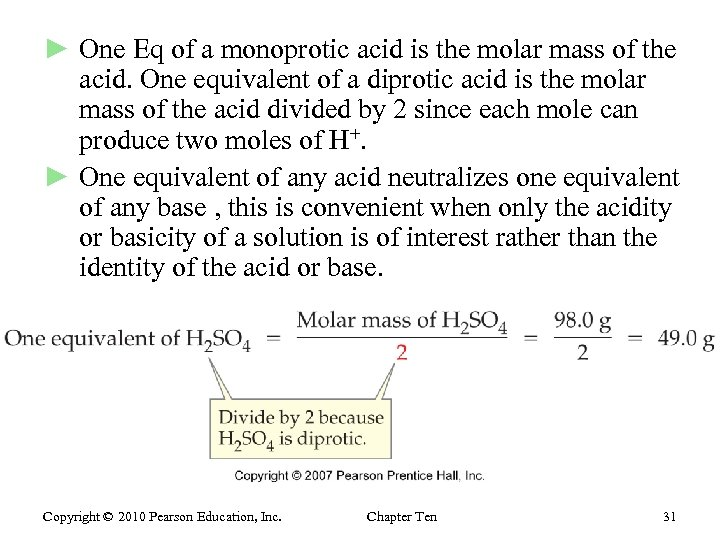► One Eq of a monoprotic acid is the molar mass of the acid. One equivalent of a diprotic acid is the molar mass of the acid divided by 2 since each mole can produce two moles of H+. ► One equivalent of any acid neutralizes one equivalent of any base , this is convenient when only the acidity or basicity of a solution is of interest rather than the identity of the acid or base. Copyright © 2010 Pearson Education, Inc. Chapter Ten 31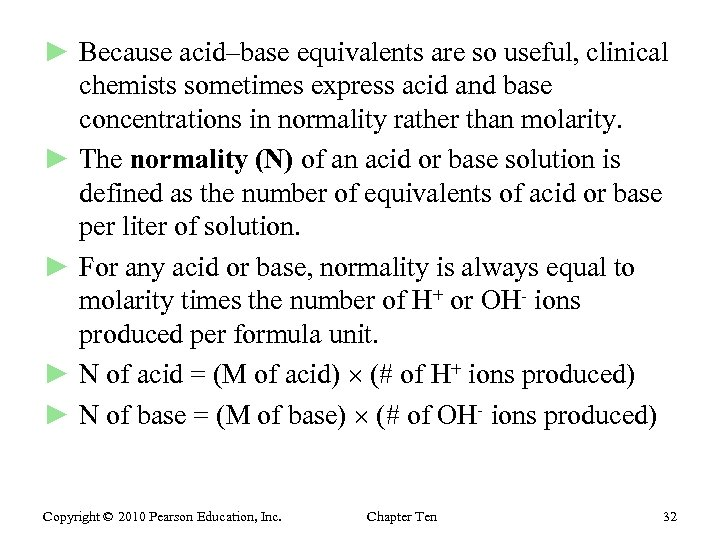► Because acid–base equivalents are so useful, clinical chemists sometimes express acid and base concentrations in normality rather than molarity. ► The normality (N) of an acid or base solution is defined as the number of equivalents of acid or base per liter of solution. ► For any acid or base, normality is always equal to molarity times the number of H+ or OH- ions produced per formula unit. ► N of acid = (M of acid) (# of H+ ions produced) ► N of base = (M of base) (# of OH- ions produced) Copyright © 2010 Pearson Education, Inc. Chapter Ten 32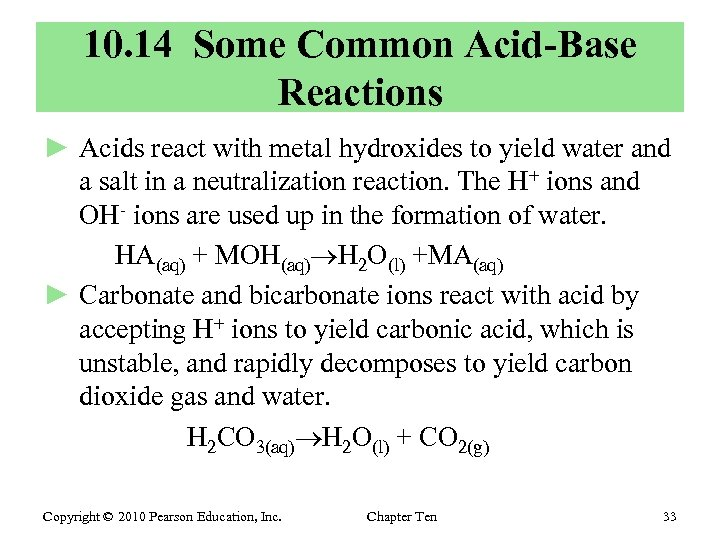10. 14 Some Common Acid-Base Reactions ► Acids react with metal hydroxides to yield water and a salt in a neutralization reaction. The H+ ions and OH- ions are used up in the formation of water. HA(aq) + MOH(aq) H 2 O(l) +MA(aq) ► Carbonate and bicarbonate ions react with acid by accepting H+ ions to yield carbonic acid, which is unstable, and rapidly decomposes to yield carbon dioxide gas and water. H 2 CO 3(aq) H 2 O(l) + CO 2(g) Copyright © 2010 Pearson Education, Inc. Chapter Ten 33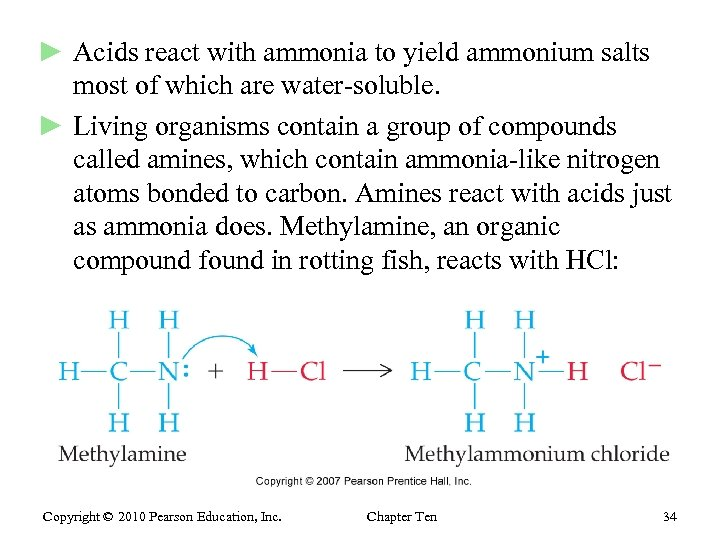► Acids react with ammonia to yield ammonium salts most of which are water-soluble. ► Living organisms contain a group of compounds called amines, which contain ammonia-like nitrogen atoms bonded to carbon. Amines react with acids just as ammonia does. Methylamine, an organic compound found in rotting fish, reacts with HCl: Copyright © 2010 Pearson Education, Inc. Chapter Ten 34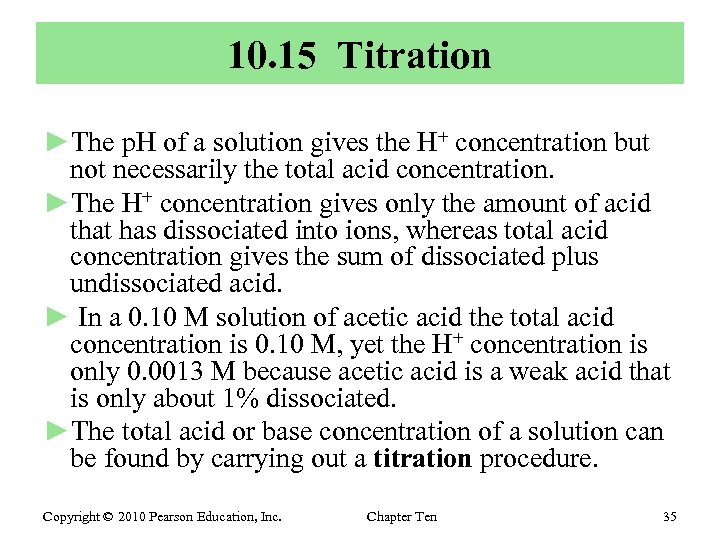10. 15 Titration ►The p. H of a solution gives the H+ concentration but not necessarily the total acid concentration. ►The H+ concentration gives only the amount of acid that has dissociated into ions, whereas total acid concentration gives the sum of dissociated plus undissociated acid. ► In a 0. 10 M solution of acetic acid the total acid concentration is 0. 10 M, yet the H+ concentration is only 0. 0013 M because acetic acid is a weak acid that is only about 1% dissociated. ►The total acid or base concentration of a solution can be found by carrying out a titration procedure. Copyright © 2010 Pearson Education, Inc. Chapter Ten 35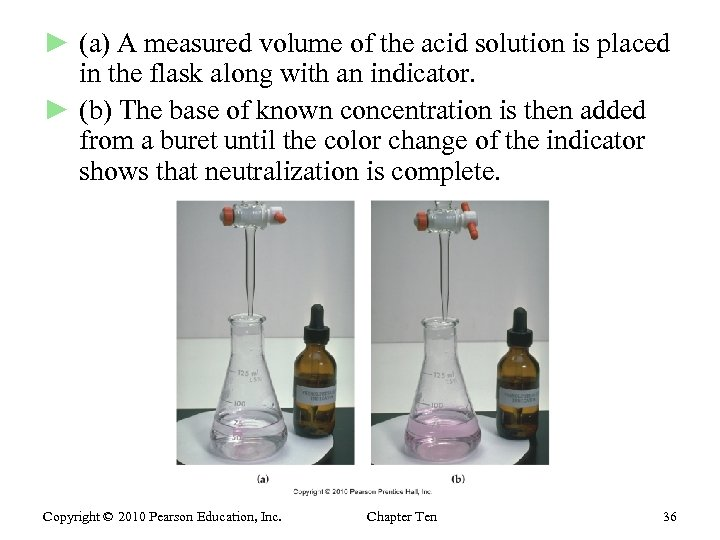► (a) A measured volume of the acid solution is placed in the flask along with an indicator. ► (b) The base of known concentration is then added from a buret until the color change of the indicator shows that neutralization is complete. Copyright © 2010 Pearson Education, Inc. Chapter Ten 36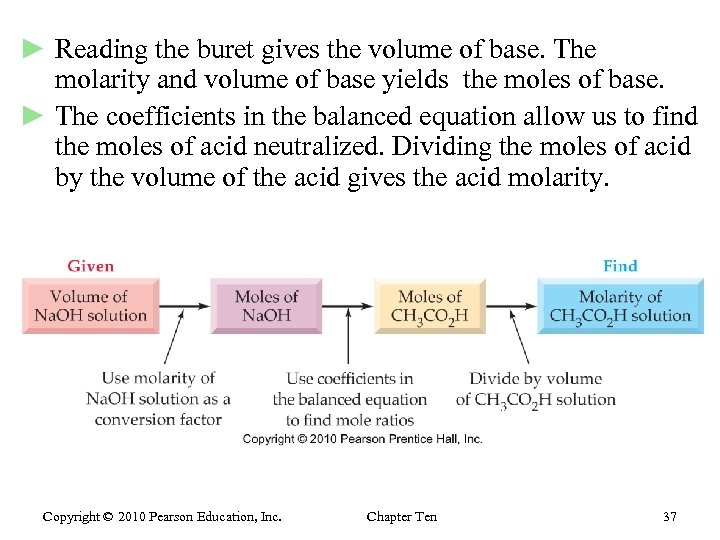► Reading the buret gives the volume of base. The molarity and volume of base yields the moles of base. ► The coefficients in the balanced equation allow us to find the moles of acid neutralized. Dividing the moles of acid by the volume of the acid gives the acid molarity. Copyright © 2010 Pearson Education, Inc. Chapter Ten 37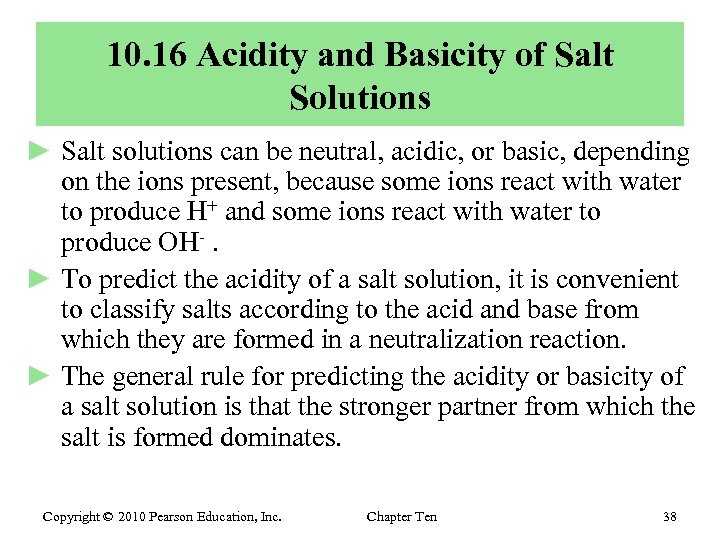10. 16 Acidity and Basicity of Salt Solutions ► Salt solutions can be neutral, acidic, or basic, depending on the ions present, because some ions react with water to produce H+ and some ions react with water to produce OH-. ► To predict the acidity of a salt solution, it is convenient to classify salts according to the acid and base from which they are formed in a neutralization reaction. ► The general rule for predicting the acidity or basicity of a salt solution is that the stronger partner from which the salt is formed dominates. Copyright © 2010 Pearson Education, Inc. Chapter Ten 38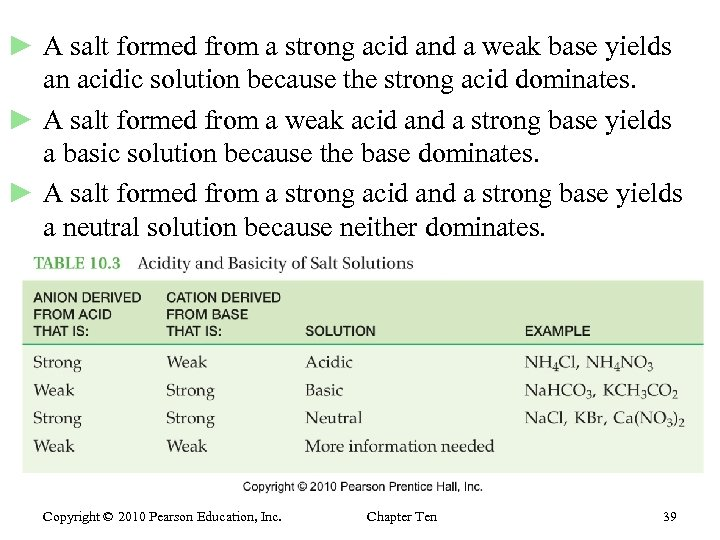► A salt formed from a strong acid and a weak base yields an acidic solution because the strong acid dominates. ► A salt formed from a weak acid and a strong base yields a basic solution because the base dominates. ► A salt formed from a strong acid and a strong base yields a neutral solution because neither dominates. Copyright © 2010 Pearson Education, Inc. Chapter Ten 39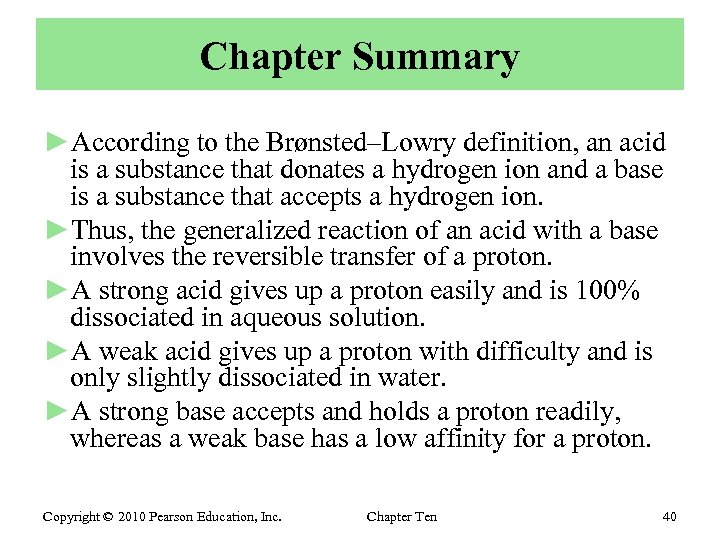Chapter Summary ►According to the Brønsted–Lowry definition, an acid is a substance that donates a hydrogen ion and a base is a substance that accepts a hydrogen ion. ►Thus, the generalized reaction of an acid with a base involves the reversible transfer of a proton. ►A strong acid gives up a proton easily and is 100% dissociated in aqueous solution. ►A weak acid gives up a proton with difficulty and is only slightly dissociated in water. ►A strong base accepts and holds a proton readily, whereas a weak base has a low affinity for a proton. Copyright © 2010 Pearson Education, Inc. Chapter Ten 40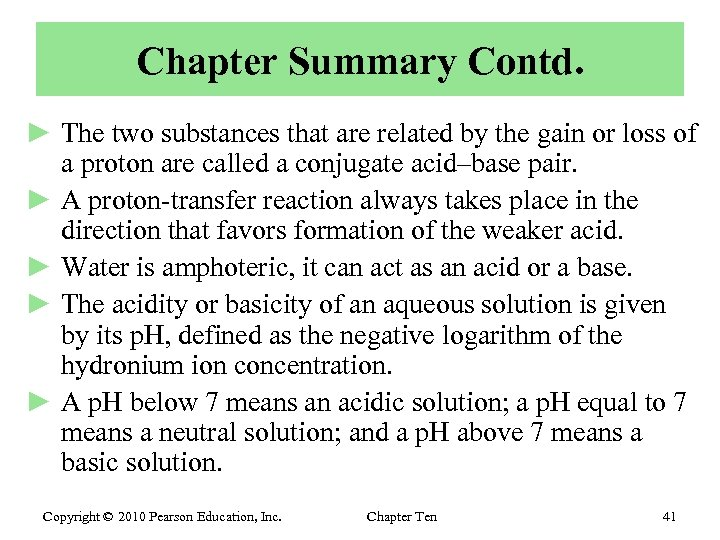Chapter Summary Contd. ► The two substances that are related by the gain or loss of a proton are called a conjugate acid–base pair. ► A proton-transfer reaction always takes place in the direction that favors formation of the weaker acid. ► Water is amphoteric, it can act as an acid or a base. ► The acidity or basicity of an aqueous solution is given by its p. H, defined as the negative logarithm of the hydronium ion concentration. ► A p. H below 7 means an acidic solution; a p. H equal to 7 means a neutral solution; and a p. H above 7 means a basic solution. Copyright © 2010 Pearson Education, Inc. Chapter Ten 41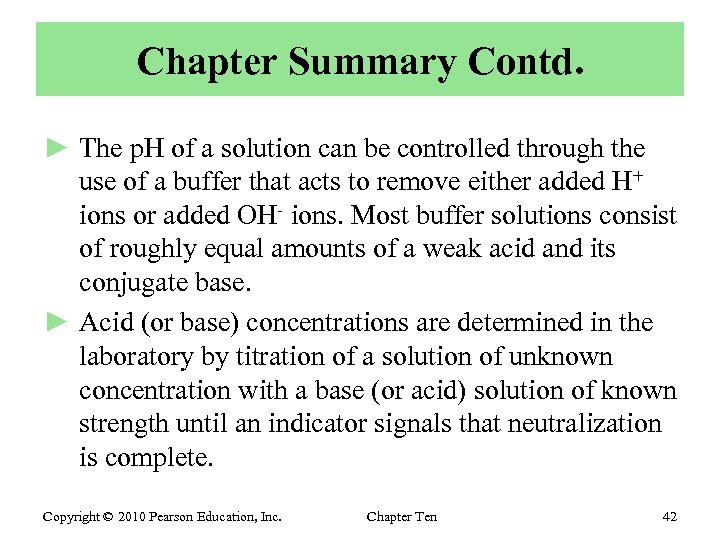Chapter Summary Contd. ► The p. H of a solution can be controlled through the use of a buffer that acts to remove either added H+ ions or added OH- ions. Most buffer solutions consist of roughly equal amounts of a weak acid and its conjugate base. ► Acid (or base) concentrations are determined in the laboratory by titration of a solution of unknown concentration with a base (or acid) solution of known strength until an indicator signals that neutralization is complete. Copyright © 2010 Pearson Education, Inc. Chapter Ten 42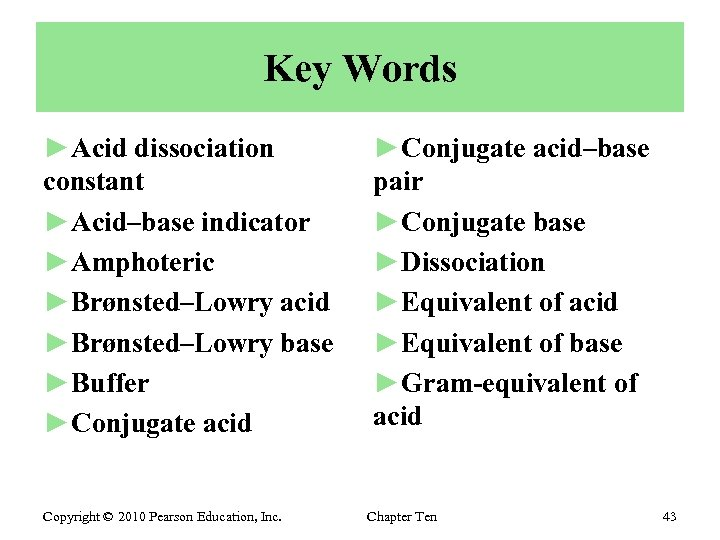Key Words ►Acid dissociation constant ►Acid–base indicator ►Amphoteric ►Brønsted–Lowry acid ►Brønsted–Lowry base ►Buffer ►Conjugate acid Copyright © 2010 Pearson Education, Inc. ►Conjugate acid–base pair ►Conjugate base ►Dissociation ►Equivalent of acid ►Equivalent of base ►Gram-equivalent of acid Chapter Ten 43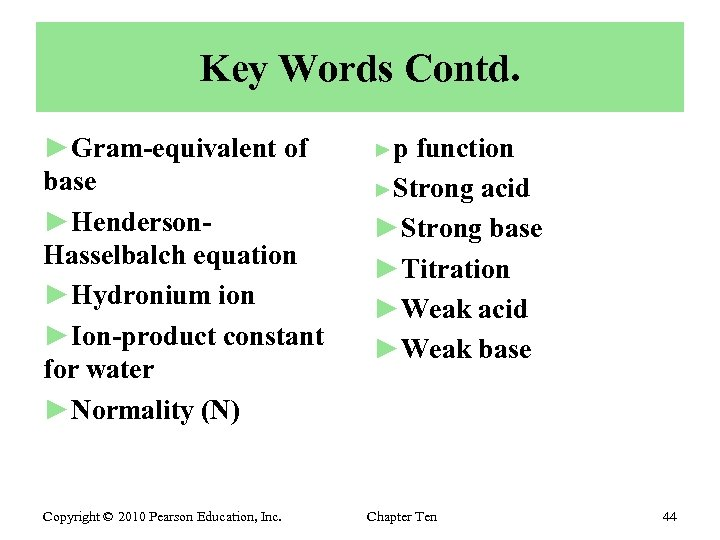Key Words Contd. ►Gram-equivalent of base ►Henderson. Hasselbalch equation ►Hydronium ion ►Ion-product constant for water ►Normality (N) Copyright © 2010 Pearson Education, Inc. ►p function ►Strong acid ►Strong base ►Titration ►Weak acid ►Weak base Chapter Ten 44End of Chapter 10 Copyright © 2010 Pearson Education, Inc. Chapter Ten 45